Updating search results...# Interactive Mini-Lessons and Simulations

Interactive lessons that provide ways for students to manipulate data and use interactive tools to gain a deeper understanding of complex topics.
354 affiliated resources

# Search Resources

View
Selected filters: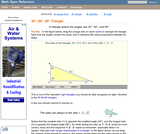Rating
5.0 stars

An interactive applet and associated web page that demonstrate the properties of a 30-60-90 triangle. The applet shows a right triangle that can be resized by dragging any vertex. As it is dragged, the remaining vertices change so that the triangle's angles remain 30 degrees, 60 degrees and 90 degrees The text on the page points out that the sides of a 30-60-90 triangle are always in the ratio of 1 : 2 : root 3 Applet can be enlarged to full screen size for use with a classroom projector. This resource is a component of the Math Open Reference Interactive Geometry textbook project at http://www.mathopenref.com.

Subject:
Geometry
Material Type:
Simulation
Provider:
Math Open Reference
Author:
John Page
02/16/2011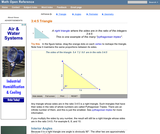Rating
5.0 stars

An interactive applet and associated web page that demonstrate the properties of a 3:4:5 triangle - one of the Pythagorean triples. The applet shows a right triangle that can be resized by dragging any vertex. As it is dragged, the remaining vertices change so that the triangle's side remain in the ration 3:4:5. The text on the page has an example of how the triangle can be used to measure a right angle on even large objects. Applet can be enlarged to full screen size for use with a classroom projector. This resource is a component of the Math Open Reference Interactive Geometry textbook project at http://www.mathopenref.com.

Subject:
Geometry
Material Type:
Simulation
Provider:
Math Open Reference
Author:
John Page
02/16/2011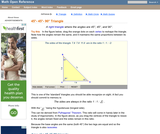Rating
3.0 stars

An interactive applet and associated web page that demonstrate the properties of a 45-45-90 isosceles right triangle. The applet shows a right triangle that can be resized by dragging any vertex. As it is dragged, the remaining vertices change so that the triangle's angles remain 45 degrees, 45 degrees and 90 degrees The text on the page points out that the sides of a 45-45-90 triangle are always in the ratio of 1 : 2 : root 2 Applet can be enlarged to full screen size for use with a classroom projector. This resource is a component of the Math Open Reference Interactive Geometry textbook project at http://www.mathopenref.com.

Subject:
Geometry
Material Type:
Simulation
Provider:
Math Open Reference
Author:
John Page
02/16/2011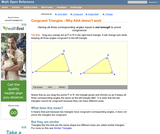Rating
0.0 stars

An interactive applet and associated web page that shows that angle-angle-angle (AAA) is not enough to prove congruence. The applet shows two triangles, one of which can be dragged to resize it, showing that although they have the same angles they are not the same size and thus not congruent. The web page describes all this and has links to other related pages. Applet can be enlarged to full screen size for use with a classroom projector. This resource is a component of the Math Open Reference Interactive Geometry textbook project at http://www.mathopenref.com.

Subject:
Geometry
Material Type:
Simulation
Provider:
Math Open Reference
Author:
John Page
02/16/2011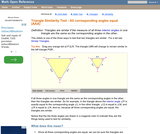Rating
4.0 stars

An interactive applet and associated web page showing how the AAA similarity test works. Two similar triangles are shown that can be resized by dragging. The other triangle adjusts to remain similar and the angle-angle-angle elements are highlighted to show how they are involved in this test of similarity. (all three interior angles congruent). The web page describes all this and has links to other related pages. Applet can be enlarged to full screen size for use with a classroom projector. This resource is a component of the Math Open Reference interactive geometry reference book project at http://www.mathopenref.com.

Subject:
Geometry
Material Type:
Simulation
Provider:
Math Open Reference
Author:
John Page
02/16/2011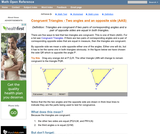Rating
3.66666666667 stars

An interactive applet and associated web page that shows how triangles that have two angles and a non-included side the same must be congruent. The applet shows two triangles, one of which can be reshaped by dragging any vertex. The other changes to remain congruent to it and the two angles and non-included side are outlined in bold to show they are the same measure and are the elements being used to prove congruence. The web page describes all this and has links to other related pages. Applet can be enlarged to full screen size for use with a classroom projector. This resource is a component of the Math Open Reference Interactive Geometry textbook project at http://www.mathopenref.com.

Subject:
Geometry
Material Type:
Simulation
Provider:
Math Open Reference
Author:
John Page
02/16/2011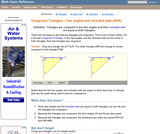Rating
0.0 stars

An interactive applet and associated web page that shows how triangles that have two angles and their included side the same must be congruent. The applet shows two triangles, one of which can be reshaped by dragging any vertex. The other changes to remain congruent to it and the two angles and the included side are outlined in bold to show they are the same measure and are the elements being used to prove congruence. The web page describes all this and has links to other related pages. Applet can be enlarged to full screen size for use with a classroom projector. This resource is a component of the Math Open Reference Interactive Geometry textbook project at http://www.mathopenref.com.

Subject:
Geometry
Material Type:
Simulation
Provider:
Math Open Reference
Author:
John Page
02/16/2011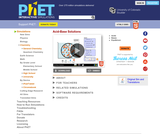Unrestricted Use
CC BY
Rating
4.6 stars

How do strong and weak acids differ? Use lab tools on your computer to find out! Dip the paper or the probe into solution to measure the pH, or put in the electrodes to measure the conductivity. Then see how concentration and strength affect pH. Can a weak acid solution have the same pH as a strong acid solution?

Subject:
Chemistry
Material Type:
Simulation
Provider:
Provider Set:
PhET Interactive Simulations
Author:
Chris Malley
Kathy Perkins
Kelly Lancaster
Patricia Loeblein
Robert Parson
09/01/2010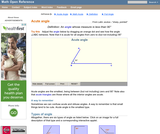Rating
0.0 stars

An interactive applet and associated web page that demonstrate acute angles (those less than 90 deg). The applet presents an angle (initially acute) that the user can adjust by dragging the end points of the line segments forming the angle. As it changes it shows the angle measure and a message that indicate which type of angle it is. There a software 'detents' that make it easy capture exact angles such as 90 degrees and 180 degrees The message and angle measures can be turned off to facilitate classroom discussion. The text on the page has links to other pages defining each angle type in depth. Applet can be enlarged to full screen size for use with a classroom projector. This resource is a component of the Math Open Reference Interactive Geometry textbook project at http://www.mathopenref.com.

Subject:
Geometry
Material Type:
Simulation
Provider:
Math Open Reference
Author:
John Page
02/16/2011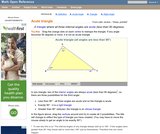Rating
0.0 stars

An interactive applet and associated web page that demonstrate the three types of triangle: acute, obtuse and right. The applet shows a triangle that is initially acute (all angles less then 90 degrees) which the user can reshape by dragging any vertex. There is a message changes in real time while the triangle is being dragged that tells if the triangle is an acute, right or obtuse triangle and gives the reason why. By experimenting with the triangle student can develop an intuitive sense of the difference between these three classes of triangle. Applet can be enlarged to full screen size for use with a classroom projector. This resource is a component of the Math Open Reference Interactive Geometry textbook project at http://www.mathopenref.com.

Subject:
Geometry
Material Type:
Simulation
Provider:
Math Open Reference
Author:
John Page
02/16/2011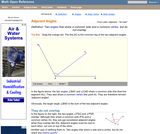Rating
3.0 stars

An interactive applet and associated web page that show the concept of adjacent angles (two angles that share a common leg). The applet shows three line segments with a common endpoint. The user can move the center one and see that the angles on both sides (the adjacent angles) of it are affected. Applet can be enlarged to full screen size for use with a classroom projector. After use in the classroom, students can access it again from any web browser at home or in the library with no login required. This resource is a component of the Math Open Reference Interactive Geometry textbook project at http://www.mathopenref.com.

Subject:
Geometry
Material Type:
Simulation
Provider:
Math Open Reference
Author:
John Page
02/16/2011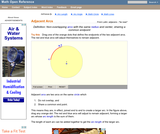Rating
0.0 stars

An interactive applet and associated web page that demonstrate the the concept of adjacent arcs. The user can 3 drag points around a circle that define the endpoints of two adjacent arcs. Web page has the properties of adjacent arcs. Applet can be enlarged to full screen size for use with a classroom projector. This resource is a component of the Math Open Reference Interactive Geometry textbook project at http://www.mathopenref.com.

Subject:
Geometry
Material Type:
Simulation
Provider:
Math Open Reference
Author:
John Page
02/16/2011Conditional Remix & Share Permitted
CC BY-NC
Rating
0.0 stars

In this module, students engage in a visual demonstration on the causes & effects of air pollutants on air quality and kinesthetic activities on particulate matter & visibility.

Subject:
Atmospheric Science
Material Type:
Lesson
Provider:
Provider Set:
Cooperative Institute for Research in Environmental Sciences (CIRES)
01/01/2014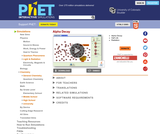Unrestricted Use
CC BY
Rating
0.0 stars

Watch alpha particles escape from a polonium nucleus, causing radioactive alpha decay. See how random decay times relate to the half life.

Subject:
Physical Science
Material Type:
Simulation
Provider:
Provider Set:
PhET Interactive Simulations
Author:
Carl Wieman
Danielle Harlow
John Blanco
Kathy Perkins
Noah Podolefsky
Ron LeMaster
Sam McKagan
07/21/2011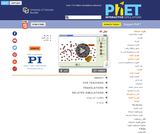Unrestricted Use
CC BY
Rating
0.0 stars

Watch alpha particles escape from a polonium nucleus, causing radioactive alpha decay. See how random decay times relate to the half life.

Subject:
Physical Science
Material Type:
Simulation
Provider:
Provider Set:
PhET Interactive Simulations
Author:
Carl Wieman
Danielle Harlow
John Blanco
Kathy Perkins
Noah Podolefsky
Ron LeMaster
Sam McKagan
09/02/2012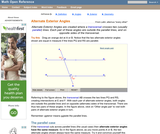Rating
0.0 stars

An interactive applet and associated web page that demonstrate the alternate exterior angles that are formed where a transversal crosses two lines. The applets shows the two possible pairs of angles alternating when in animation mode. By dragging the three lines, it can be seen that the angles are congruent only when the lines are parallel. When not in animated mode, there is a button that alternates the two pairs of angles. The text on the page discusses the properties of the angle pairs both in the parallel and non-parallel cases. Applet can be enlarged to full screen size for use with a classroom projector. This resource is a component of the Math Open Reference Interactive Geometry textbook project at http://www.mathopenref.com.

Subject:
Geometry
Material Type:
Simulation
Provider:
Math Open Reference
Author:
John Page
02/16/2011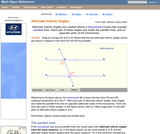Rating
0.0 stars

An interactive applet and associated web page that demonstrate the alternate interior angles that are formed where a transversal crosses two lines. The applets shows the two possible pairs of angles alternating when in animation mode. By dragging the three lines, it can be seen that the angles are congruent only when the lines are parallel. When not in animated mode, there is a button that alternates the two pairs of angles. The text on the page discusses the properties of the angle pairs both in the parallel and non-parallel cases. Applet can be enlarged to full screen size for use with a classroom projector. This resource is a component of the Math Open Reference Interactive Geometry textbook project at http://www.mathopenref.com.

Subject:
Geometry
Material Type:
Simulation
Provider:
Math Open Reference
Author:
John Page
02/16/2011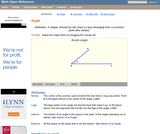Rating
0.0 stars

An interactive applet and associated web page that introduce the concept of an angle. An angle made from two line segments is shown that the user can adjust by dragging the end points of the segments. In real time, as the angles is changed by the user, the angle measure in degrees is shown and a message telling what type of angle it currently is: acute, right, obtuse, reflex or straight. Applet can be enlarged to full screen size for use with a classroom projector. This resource is a component of the Math Open Reference Interactive Geometry textbook project at http://www.mathopenref.com.

Subject:
Geometry
Material Type:
Simulation
Provider:
Math Open Reference
Author:
John Page
02/16/2011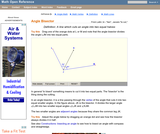Rating
0.0 stars

An interactive applet and associated web page that demonstrate the bisector of an angle. An angle is shown using two line segments that can be dragged to change the angle measure. The angle is bisected by a line which moves while dragging to always divide the angle into two equal angles. The angle measures can be turned off for class discussions. Applet can be enlarged to full screen size for use with a classroom projector. This resource is a component of the Math Open Reference Interactive Geometry textbook project at http://www.mathopenref.com.

Subject:
Geometry
Material Type:
Simulation
Provider:
Math Open Reference
Author:
John Page
02/16/2011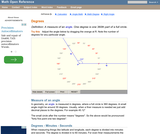Rating
0.0 stars

An interactive applet that acts as a 'digital manipulative' for explaining angles measured in degrees. The applet has an angle formed from two segment that can be dragged around in a circle. The angle measure is shown against a 'clock face' calibrated in degrees. The measures can be turned off for class angle estimation discussions. Applet can be enlarged to full screen size for use with a classroom projector. This resource is a component of the Math Open Reference Interactive Geometry textbook project at http://www.mathopenref.com.

Subject:
Geometry
Material Type:
Simulation
Provider:
Math Open Reference
Author:
John Page
02/16/2011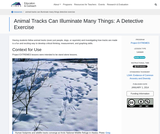Conditional Remix & Share Permitted
CC BY-NC
Rating
0.0 stars

Having students follow animal tracks (even just people, dogs, or squirrels) and investigating how tracks are made is a fun and exciting way to develop critical thinking, measurement, and graphing skills.

Subject:
Applied Science
Mathematics
Material Type:
Lesson
Provider:
Provider Set:
Cooperative Institute for Research in Environmental Sciences (CIRES)
01/01/2014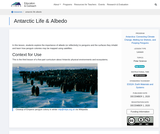Conditional Remix & Share Permitted
CC BY-NC
Rating
0.0 stars

In this lesson, students explore the importance of albedo (or reflectivity) to penguins and the surfaces they inhabit and learn how penguin colonies may be mapped using satellites.

Subject:
Atmospheric Science
Material Type:
Lesson
Provider:
Provider Set:
Cooperative Institute for Research in Environmental Sciences (CIRES)
12/01/2020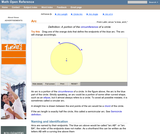Rating
0.0 stars

An interactive applet and associated web page that demonstrate the concept of an arc. The applet shows a circle with part of it highlighted to identify the arc. Each endpoint of the arc can be dragged to resize it. The web page has definitions and links to the properties of an arc. Applet can be enlarged to full screen size for use with a classroom projector. This resource is a component of the Math Open Reference Interactive Geometry textbook project at http://www.mathopenref.com.

Subject:
Geometry
Material Type:
Simulation
Provider:
Math Open Reference
Author:
John Page
02/16/2011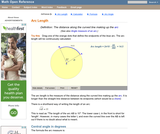Rating
0.0 stars

An interactive applet and associated web page that demonstrate the concept of arc length. The applet shows a circle with part of its circumference highlighted and the central angle shown. As the user drags either end of the arc it is redrawn and the calculation for arc length changes as you drag. The text on the web page gives the formula for calculating the arc length. Applet can be enlarged to full screen size for use with a classroom projector. This resource is a component of the Math Open Reference Interactive Geometry textbook project at http://www.mathopenref.com.

Subject:
Geometry
Material Type:
Simulation
Provider:
Math Open Reference
Author:
John Page
02/16/2011Conditional Remix & Share Permitted
CC BY-NC
Rating
0.0 stars

In this middle school and high school unit, students engage with 360° virtual reality tours, authentic Arctic datasets, and app-based labs to construct models and explanations for the unit driving question, "Why might the Arctic be warming twice as fast as the rest of the world?"

Subject:
Applied Science
Physical Science
Material Type:
Unit of Study
Provider:
Provider Set:
Cooperative Institute for Research in Environmental Sciences (CIRES)
06/25/2020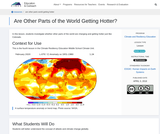Conditional Remix & Share Permitted
CC BY-NC
Rating
0.0 stars

In this lesson, students investigate whether other parts of the world are changing and getting hotter just like Colorado.

Subject:
Environmental Science
Atmospheric Science
Material Type:
Lesson
Provider:
Provider Set:
Cooperative Institute for Research in Environmental Sciences (CIRES)
04/05/2018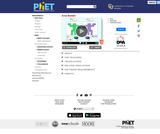Unrestricted Use
CC BY
Rating
4.33333333333 stars

Create your own shapes using colorful blocks and explore the relationship between perimeter and area. Compare the area and perimeter of two shapes side-by-side. Challenge yourself in the game screen to build shapes or find the area of funky figures. Try to collect lots of stars!

Subject:
Mathematics
Material Type:
Simulation
Provider:
Provider Set:
PhET Interactive Simulations
Author:
Amanda McGarry
Ariel Paul
Bryce Gruneich
John Blanco
Kathy Perkins
01/08/2018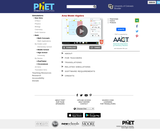Unrestricted Use
CC BY
Rating
4.0 stars

Build rectangles of various sizes and relate multiplication to area. Discover new strategies for multiplying algebraic expressions. Use the game screen to test your multiplication and factoring skills!

Subject:
Algebra
Material Type:
Diagram/Illustration
Game
Interactive
Simulation
Provider:
Provider Set:
PhET Interactive Simulations
Author:
Ariel Paul
Diana Lopez Tavares (artwork)
Jonathan Olson (developer)
Karina Hensberry
Kathy Perkins
Mariah Hermsmeyer (artwork)
Susan Miller
05/09/2018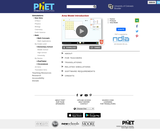Unrestricted Use
CC BY
Rating
4.0 stars

Build rectangles of various sizes and relate multiplication to area. Partition a rectangle into two areas to discover the distributive property.

Subject:
Elementary Education
Algebra
Numbers and Operations
Material Type:
Diagram/Illustration
Game
Interactive
Simulation
Provider:
Provider Set:
PhET Interactive Simulations
Author:
Ariel Paul
Diana Lopez Tavares (artwork)
Jonathan Olson (developer)
Karina Hensberry
Kathy Perkins
Mariah Hermsmeyer (artwork)
Susan Miller
05/15/2018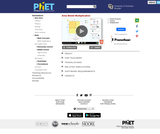Unrestricted Use
CC BY
Rating
0.0 stars

Build rectangles of various sizes and relate multiplication to area. Discover new strategies for multiplying large numbers. Use the game screen to test your problem solving strategies!

Subject:
Elementary Education
Algebra
Numbers and Operations
Material Type:
Diagram/Illustration
Game
Interactive
Simulation
Provider:
Provider Set:
PhET Interactive Simulations
Author:
Ariel Paul
Diana Lopez Tavares (artwork)
Jonathan Olson (developer)
Karina Hensberry
Kathy Perkins
Mariah Hermsmeyer (artwork)
Susan Miller
05/15/2018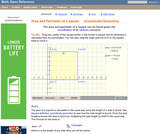Rating
0.0 stars

An interactive applet and associated web page showing how to find the area and perimeter of a square from the coordinates of its vertices. The square can be either parallel to the axes or rotated. The grid and coordinates can be turned on and off. The area and perimeter calculation can be turned off to permit class exercises and then turned back on the verify the answers. The applet can be printed as it appears on the screen to make handouts. The web page has a full description of the method for determining area and perimeter, a worked example and has links to other pages relating to coordinate geometry. Applet can be enlarged to full screen size for use with a classroom projector. This resource is a component of the Math Open Reference Interactive Geometry textbook project at http://www.mathopenref.com.

Subject:
Geometry
Material Type:
Simulation
Provider:
Math Open Reference
Author:
John Page
02/16/2011Rating
0.0 stars

An interactive applet and associated web page that demonstrate the area of a circle. A circle is shown with a point on the circumference that can be dragged to resize the circle. As the circle is resized, the radius and the area computation is shown changing in real time. The radius and formula can be hidden for class discussion. Applet can be enlarged to full screen size for use with a classroom projector. This resource is a component of the Math Open Reference Interactive Geometry textbook project at http://www.mathopenref.com.

Subject:
Geometry
Material Type:
Simulation
Provider:
Math Open Reference
Author:
John Page
02/16/2011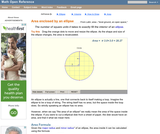Rating
0.0 stars

An interactive applet and associated web page that demonstrate the area of an ellipse. The major and minor axes can be dragged and the area is continuously recalculated. The ellipse has a grid inside it so that students can estimate the area and compare the result to the calculated one. The web page has the formula for the area calculation. The web page also has links to other pages defining the various properties of an ellipse and to some ellipse constructions. Applet can be enlarged to full screen size for use with a classroom projector. This resource is a component of the Math Open Reference Interactive Geometry textbook project at http://www.mathopenref.com.

Subject:
Geometry
Material Type:
Simulation
Provider:
Math Open Reference
Author:
John Page
02/16/2011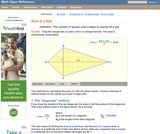Rating
0.0 stars

An interactive applet and associated web page that deals with the area of a kite, (a quadrilateral with two distinct pairs of equal adjacent sides). The applet shows a kite and the user can reshape it by dragging any vertex. The other vertices move automatically to ensure it always remains a kite. As the vertices are dragged, the area is continuously recalculated and displayed. The kite is filled with a grid of unit squares so that the students can estimate the area. The on-screen calculation can be hidden until the estimates are done. The web page lists two different ways to compute the area of a kite. Applet can be enlarged to full screen size for use with a classroom projector. This resource is a component of the Math Open Reference Interactive Geometry textbook project at http://www.mathopenref.com.

Subject:
Geometry
Material Type:
Simulation
Provider:
Math Open Reference
Author:
John Page
02/16/2011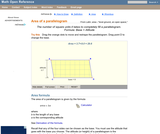Rating
0.0 stars

A web page and interactive applet showing the ways to calculate the area of a parallelogram. The user can drag the vertices of the parallelogram and the other points change automatically to ensure it remains a parallelogram. A grid inside the shape allows students to estimate the area visually, then check against the actual computed area, which is continuously recomputed and displayed. The text on the page gives three different ways to calculate the area with a formula for each. The applet uses one of the methods to compute the area in real time, so it changes as the rhombus is reshaped with the mouse. A companion page is http://www.mathopenref.com/parallelogram.html showing the definition and properties of a parallelogram Applet can be enlarged to full screen size for use with a classroom projector. This resource is a component of the Math Open Reference Interactive Geometry textbook project at http://www.mathopenref.com.

Subject:
Geometry
Material Type:
Simulation
Provider:
Math Open Reference
Author:
John Page
02/16/2011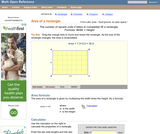Rating
0.0 stars

A web page and interactive applet showing the ways to calculate the area of a rectangle. The user can drag the vertices of the rectangle and the other points change automatically to ensure it remains a rectangle. A grid inside the shape allows students to estimate the area visually, then check against the actual computed area. The text on the page gives three different ways to calculate the area with a formula for each. The applet uses one of the methods to compute the area in real time, so it changes as the rectangle is reshaped with the mouse. A companion page is http://www.mathopenref.com/rectangle.html showing the definition and properties of a rectangle Applet can be enlarged to full screen size for use with a classroom projector. This resource is a component of the Math Open Reference Interactive Geometry textbook project at http://www.mathopenref.com.

Subject:
Geometry
Material Type:
Simulation
Provider:
Math Open Reference
Author:
John Page
02/16/2011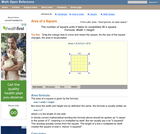Rating
0.0 stars

An interactive applet and associated web page that demonstrate the area of a square. The applet shows a square with all vertices draggable. As you drag any one, the area id continuously calculated and shown on the applet. The square is filled with a unit grid to allow class estimation of area. The displayed calculation can be turned off. Applet can be enlarged to full screen size for use with a classroom projector. This resource is a component of the Math Open Reference Interactive Geometry textbook project at http://www.mathopenref.com.

Subject:
Geometry
Material Type:
Simulation
Provider:
Math Open Reference
Author:
John Page
02/16/2011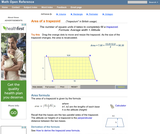Rating
2.0 stars

A web page and interactive applet showing the ways to calculate the area of a trapezoid. The user can drag the vertices of the trapezoid and the other points change automatically to ensure it remains a trapezoid. A grid inside the shape allows students to estimate the area visually, then check against the actual computed area. The text on the page gives three different ways to calculate the area with a formula for each. The applet uses one of the methods to compute the area in real time, so it changes as the trapezoid is reshaped with the mouse. A companion page is http://www.mathopenref.com/trapezoid.html showing the definition and properties of a trapezoid. Applet can be enlarged to full screen size for use with a classroom projector. This resource is a component of the Math Open Reference Interactive Geometry textbook project at http://www.mathopenref.com.

Subject:
Geometry
Material Type:
Simulation
Provider:
Math Open Reference
Author:
John Page
02/16/2011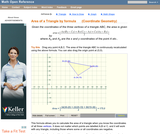Rating
0.0 stars

An interactive applet and associated web page that calculate the area of a triangle using the formula method in coordinate geometry. The applet has a triangle with draggable vertices. As you drag them the triangle's area is recalculated from the vertex coordinates using the formula. The grid and coordinates can be turned on and off. The area calculation can be turned off to permit class exercises and then turned back on the verify the answers. The applet can be printed as it appears on the screen to make handouts. The web page has a full description of the method for determining area using the formula method, a worked example and has links to other pages relating to coordinate geometry. Applet can be enlarged to full screen size for use with a classroom projector. This resource is a component of the Math Open Reference Interactive Geometry textbook project at http://www.mathopenref.com.

Subject:
Geometry
Material Type:
Simulation
Provider:
Math Open Reference
Author:
John Page
02/16/2011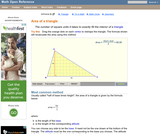Rating
0.0 stars

An interactive applet and associated web page that explain the area of a triangle. The applet shows a triangle that can be reshaped by dragging any vertex. As it changes, the area is continually recalculated using the 'half base times height' method. The triangle has a fixed square grid in its interior that can be used to visually estimate the area for later correlation with the calculated value. The calculation can be hidden while estimation is in progress. The text page has links to a similar page that uses Heron's Formula to compute the area. Applet can be enlarged to full screen size for use with a classroom projector. This resource is a component of the Math Open Reference Interactive Geometry textbook project at http://www.mathopenref.com.

Subject:
Geometry
Material Type: